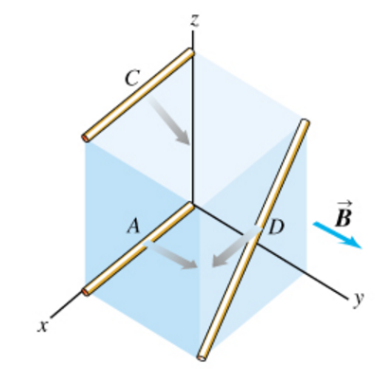# Problem: The cube shown in the figure below, 50.0 cm on a side, is in a uniform magnetic field of B = 0.125 T , directed along the positive y-axis. Wires A, C, and D move in the directions indicated, each with a speed of 0.370 m/s . (Wire A moves parallel to the xy-plane, C moves at an angle of 45.0° below the xy-plane, and D moves parallel to the xz-plane.) a. What is the potential difference between the ends of wire A? b. What is the potential difference between the ends of wire C? c. What is the potential difference between the ends of wire D?

###### FREE Expert Solution

Potential difference:

$\overline{){\mathbf{V}}{\mathbf{=}}{\mathbf{v}}{\mathbf{B}}{\mathbf{l}}{\mathbf{s}}{\mathbf{i}}{\mathbf{n}}{\mathbf{\theta }}}$

θ is the angle between the v and B vectors.

85% (398 ratings)###### Problem Details

The cube shown in the figure below, 50.0 cm on a side, is in a uniform magnetic field of B = 0.125 T , directed along the positive y-axis. Wires A, C, and D move in the directions indicated, each with a speed of 0.370 m/s . (Wire A moves parallel to the xy-plane, C moves at an angle of 45.0° below the xy-plane, and D moves parallel to the xz-plane.)

a. What is the potential difference between the ends of wire A?

b. What is the potential difference between the ends of wire C?

c. What is the potential difference between the ends of wire D?